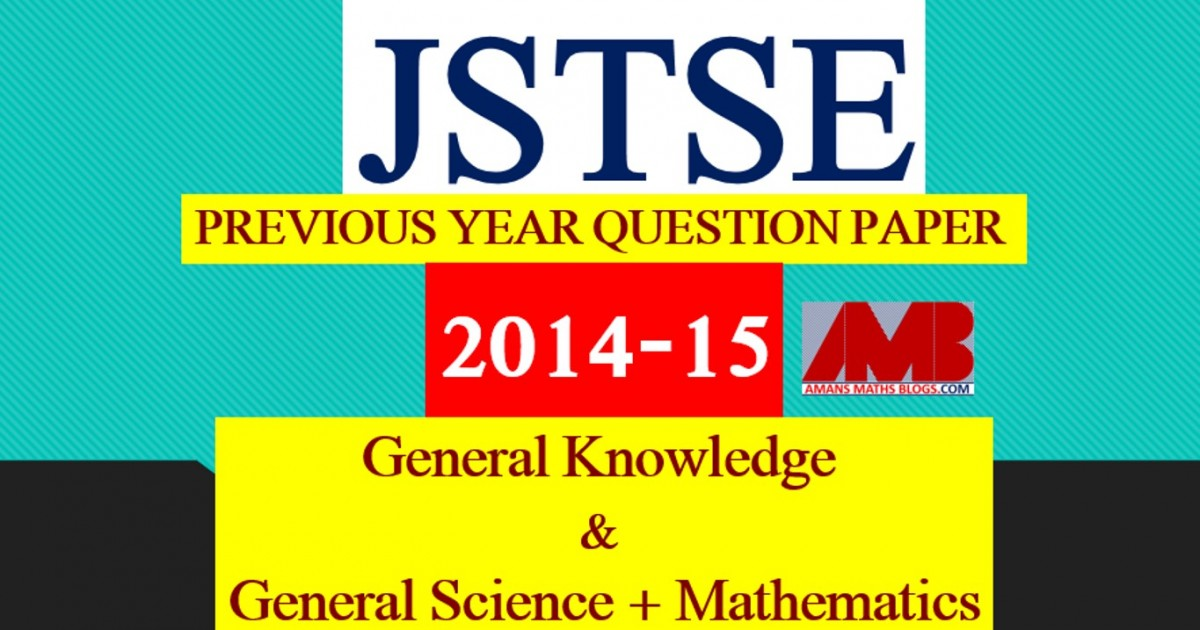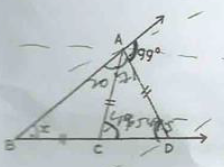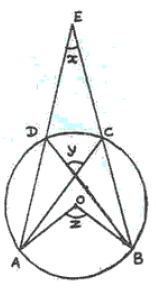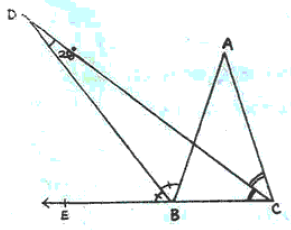Saturday, December 4, 2021
Home > download jstse papers > JSTSE PREVIOUS YEAR QUESTION PAPER 2014-15

# JSTSE PREVIOUS YEAR QUESTION PAPER 2014-15# JSTSE PREVIOUS YEAR QUESTION PAPER 2014-15

Junior Science Talent Search Examination (JSTSE) is conducted by Science Branch of DTE of Education, Delhi every year usually in the month of January for the students of recognized schools of Delhi. In this post, you will get JSTSE Previous Year Question Paper 2014-15.

JSTSE
Previous Year Question Papers
2007-082008-092009-102010-112011-12
2012-132013-142014-152015-162016-17
2018-19PhysicsChemistryMathsBiology

In JSTSE, there are two papers: Paper 1 : General Knowledge and Paper 2 :  General Science & Mathematics.

The eligibility of JSTSE exam is any students of recognizing school studying in Class 9th in Govt/Public/KV/Navodaya etc and must have secured 65% marks in Class 8th. In JSTSE, there are 150 scholarships for JSTSE per year.

Read More : 129 Maths Short Tricks

## General Science + Mathematics

JSTSE Previous Year Question Papers 2014-15 Ques No 1:

If abc = 1, then the value of 1/(1 + a + b-1) + 1/(1 + b + c-1) + 1/(1 + c + a-1) is

Options:

A. 0

B. -1

C. 1

D. 1 + a + ab

JSTSE Previous Year Question Paper 2014-15 Ques No 2:

Factors of (x4 + x2 + 1) are

Options:

A. (x2 + x + 1)(x2 + x – 1)

B. (x2 – x + 1)(x2 + x + 1)

C. (x2 – x + 1)(x2 + x – 1)

D. (x2 + 1)(x2 – 1)

JSTSE Previous Year Question Papers 2014-15 Ques No 3:

Ajay wanted to type first 180 natural numbers. Find the number of times he had to press the numbered keys

Options:

A. 416

B. 430

C. 432

D. 448

JSTSE Previous Year Question Paper 2014-15 Ques No 4:

If a = 4√2/(√2 + 1), then the value of (a + 2)/(a – 2) – (a + 2√2)/(a – √2) is

Options:

A. 12 + 8√2

B. 12 – 8√2

C. 2

D. -2

JSTSE Previous Year Question Papers 2014-15 Ques No 5:

If 52x-1 – 100 = 25x-1, then the value of 6x is

Options:

A. 6

B. 1/6

C. 36

D. 1/36

JSTSE Previous Year Question Paper 2014-15 Ques No 6:

If 3a – 2b + c = 0, then the value of 9a2 – 4b2 + c2 + 6ac is

Options:

A. -1

B. 1

C. 2

D. 0

JSTSE Previous Year Question Papers 2014-15 Ques No 7:

If (√3 + 2√5)/(√3 – 2√5) = b + a√15, then the value of a and b are

Options:

A. a = -23/17 and b = 4/17

B. a = 23/17 and b = 4/17

C. a = -4/17 and b = -23/17

D. a = -4/17 and b = 23/17

JSTSE Previous Year Question Paper 2014-15 Ques No 8:

If x = 3 + 31/3 + 32/3, then the value of x3 – 9x2 + 18x – 12 is

Options:

A. 0

B. -1

C. 1

D. 2

JSTSE Previous Year Question Papers 2014-15 Ques No 9:

In figure, AC = CB  = AD, then the value of x isOptions:

A. 49.5 degree

B. 30 degree

C. 33 degree

D. 49 degree

JSTSE Previous Year Question Paper 2014-15 Ques No 10:

One interior angle of a regular polygon is 165 degree, then the number of the sides of the polygon is

Options:

A. 15

B. 25

C. 24

D. 18

JSTSE Previous Year Question Papers 2014-15 Ques No 11:

If (9n x 32 x (3-n/2)-2 – (27)n) / (33m x 23) = 1/27, then the value of (m – n) is

Options:

A. -1

B. 1

C. 2

D. -2

JSTSE Previous Year Question Paper 2014-15 Ques No 12:

If √(3/5)1-2x = 4 17/27, then the value of x is

Options:

A. -2/7

B. 2/7

C. -7/2

D. 7/2

JSTSE Previous Year Question Papers 2014-15 Ques No 13:

The sum of five consecutive odd natural numbers is 65. The sum of the extreme numbers is

Options:

A. 24

B. 26

C. 28

D. 30

JSTSE Previous Year Question Paper 2014-15 Ques No 14:

In figure, O is the centre of the circle, thenOptions:

A. x + y + z = 180 degree

B. z = 2x + y

C. y = z + x

D. z = x + y

JSTSE Previous Year Question Papers 2014-15 Ques No 15:

The value of 0.2 + 0.23 is

Options:

A. 0.43

B. 0.43

C. 0.45

D. 0.45

JSTSE Previous Year Question Paper 2014-15 Ques No 16:

If (5x + 8y) / (6x – 7y) = 5 4/9, then the value of x/y is

Options:

A. 5/3

B. 3/5

C. -5/3

D. -3/5

JSTSE Previous Year Question Papers 2014-15 Ques No 17:

The mean of 5 observations is 15. If the mean of first three observations is 14 and the mean of the last three observation is 17, then the third observation is

Options:

A. 18

B. 19

C. 17

D. 20

JSTSE Previous Year Question Paper 2014-15 Ques No 18:

The area of a semi-circle is 308 m2, then the perimeter of the semi-circle is

Options:

A. 44 m

B. 72 m

C. 58 m

D. 88 m

JSTSE Previous Year Question Papers 2014-15 Ques No 19:

If x = (3m – 4)/5, y = (m – 7)/3 and x + y = 13/3, then the value of m is

Options:

A. 6

B. 8

C. -8

D. -4

JSTSE Previous Year Question Paper 2014-15 Ques No 20:

If the numbers 28, 25, 20, 19, 15, x, 10, 7, 6 are in ascending order and their median is then the mean is

Options:

A. 14.4

B. 15.4

C. 16.4

D. 14.6

JSTSE Previous Year Question Papers 2014-15 Ques No 21:

In the figure, CD and BD are bisectors ∠C = ∠ABE respectively. If ∠BDC = 28 degree, then the value of (∠ABC + ∠ACB) isOptions:

A. 28 degree

B. 56 degree

C. 84 degree

D. 124 degree

JSTSE Previous Year Question Papers 2014-15 Ques No 22:

The area of the square whose the sum of its diagonals is 100 cm, is

Options:

A. 5000 cm2

B. 125 cm2

C. 100√2 cm2

D. 1250 cm2

JSTSE Previous Year Question Paper 2014-15 Ques No 23:

Travelling at (4/5)th of his usual speed, a man is 15 minutes late. His usual time to cover the same distance is

Options:

A. 45 minutes

B. 60 minutes

C. 75 minutes

D. 90 minutes

JSTSE Previous Year Question Papers 2014-15 Ques No 24:

The equations 1/x + 1/y = 15 and 1/x – 1/y = 5 are such that ax = 1 and by = 1. The values of a and b respectively are:

Options:

A. 10, 5

B. 10, -5

C. -5, 10

D. 5, 10

JSTSE Previous Year Question Paper 2014-15 Ques No 25:

A conical tent is 12 m high and the radius of its base is 9 m. What is the cost of canvas required to make the tent, if the cost of 1m2 canvas Rs. 14?

Options:

A. Rs. 5840

B. Rs. 5940

C. Rs. 4950

D. Rs. 5960

JSTSE Previous Year Question Papers 2014-15 Ques No 26:

The perimeter of a rectangle whose length is 60 cm and the a diagonal is 61 cm is

Options:

A. 120 cm

B. 242 cm

C. 121 cm

D. 142 cm

JSTSE Previous Year Question Paper 2014-15 Ques No 27:

The coefficient of x2 in (x + 3)(x – 5)(x + 7) is

Options:

A. 28

B. 1

C. -5

D. 5

JSTSE Previous Year Question Papers 2014-15 Ques No 28:

The decimal expansion of 987/10500 is

Options:

A. 0.976

B. 0.094

C. 0.940

D. 0.0094

JSTSE Previous Year Question Paper 2014-15 Ques No 29:

If (x + 3) and (x + 2) are factors of 2x3 + mx2 + 7x + n, then the values of m and n are

Options:

A. m = 9, n = -6

B. m = -9, n = 6

C. m = -9, n = -6

D. m = 9, n = 6

JSTSE Previous Year Question Papers 2014-15 Ques No 30:

In the figure, ABCD is a cyclic quadrilateral and ∠ADC = 80 degree, ∠ACD = 50 degree, then the value of ∠CBD isOptions:

A. 60 degree

B. 130 degree

C. 50 degree

D. 40 degree

JSTSE
Previous Year Question Papers
2007-082008-092009-102010-112011-12
2012-132013-142014-152015-162016-17
2018-19PhysicsChemistryMathsBiology
AMBiPi
error: Content is protected !!